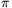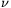Mathematical and Physical Journal
for High Schools
Issued by the MATFUND Foundation
 Already signed up? New to KöMaL?

#Problem P. 4422. (February 2012)

P. 4422. A pion (+) is a subatomic particle the mass of which is 273 times as big as that of an electron. One of its possible decay mode is a decay into a positron (e+) and an electron-neutrino (e):. What is the minimum speed of that pion which decays such that the positron and the neutrino will have perpendicular velocities? (Assume that the rest mass of the neutrino is 0, so it is a particle for which the relation between its energy and its linear momentum is E=pc.)

(6 pont)

Deadline expired on March 12, 2012.

Sorry, the solution is available only in Hungarian. Google translation

Megoldás. $\displaystyle v_{\pi}^{\rm min}=\frac{c}{\sqrt{2}}\approx 0{,}7\,c=2{,}1\cdot 10^8~\frac{\rm m}{\rm s}.$

### Statistics:

 21 students sent a solution. 6 points: Barta Szilveszter Marcell, Bolgár Dániel, Czipó Bence, Dinev Georgi, Garami Anna, Jenei Márk, Juhász Péter, Koncz Gabriella, Kovács 444 Áron, Laczkó Zoltán Balázs, Papp Roland, Szabó 928 Attila, Ürge László. 5 points: Machó Bónis, Táczi István. 4 points: 3 students. 3 points: 1 student. 1 point: 1 student. 0 point: 1 student.

Problems in Physics of KöMaL, February 2012